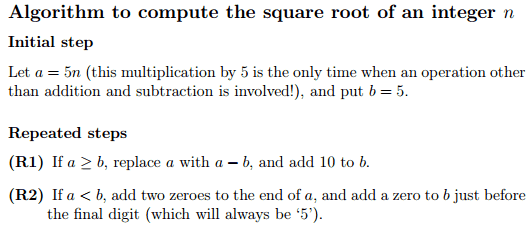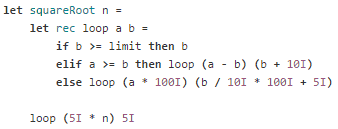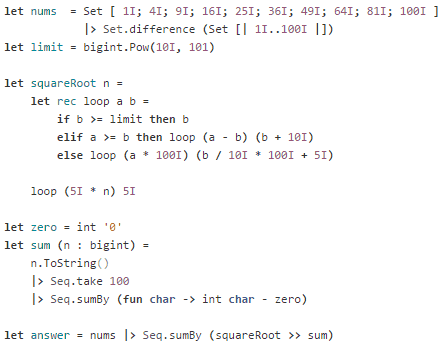# Project Euler – Problem 80 Solution

#### Problem

It is well known that if the square root of a natural number is not an integer, then it is irrational. The decimal expansion of such square roots is infinite without any repeating pattern at all.

The square root of two is 1.41421356237309504880…, and the digital sum of the first one hundred decimal digits is 475.

For the first one hundred natural numbers, find the total of the digital sums of the first one hundred decimal digits for all the irrational square roots.

#### Solution

(see full solution here).

The premise of the problem itself is fairly straight forward, the challenge here is down to the way floating points are implemented on computers which lacks the precision necessary to solve this problem. So the bulk of the research went into finding a way to generate an arbitrary number of digits of a square root.

As usual, Wikipedia has plenty to offer and the easiest solution implementation wise is this solution by Frazer Jarvis.which translates to:The rest is really straight forward, with the only tricky thing being the conversion from char to int since this returns the internal integer value instead – e.g. int ‘0’ => 48 and int ‘1’ => 49 – hence the need for some hackery in the sum function.

Here is the full solution:The solution took 95ms to complete on my machine.